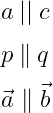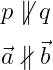# How to write a parallel symbol(∥) in LaTeX?

Geometrically, when two straight lines are at zero-degree angles to each other, they are called parallel to each other. This parallel symbol is made up of two vertical bars.

 Properties Value Symbol Parallel Package Default Command \parallel Argument No Example \parallel → ||

In latex, \|, ||, and \parallel command are used to represent two straight lines when they are parallel.

\documentclass{article}
\begin{document}
$$a \: || \: c$$
$$p \: \| \: q$$
$$\vec{a} \parallel \vec{b}$$
\end{document}

Output :If the straight lines are not parallel to each other, you can use the not parallel symbol.

\documentclass{article}
\usepackage{amssymb}
\begin{document}
$$p \not\parallel q$$
$$\vec{a} \nparallel \vec{b}$$
\end{document}

Output :You may have noticed in the program above that \not \parallel and \nparallel commands are used for parallel symbols. However, for \nparallel command, you must first install amssymb package.

And it is best practice to use \nparallel commands instead of \not\parallel commands for not parallel symbols.#### Md Jidan Mondal

LaTeX expert with over 10 years of experience in document preparation and typesetting. Specializes in creating professional documents, reports, and presentations using LaTeX.